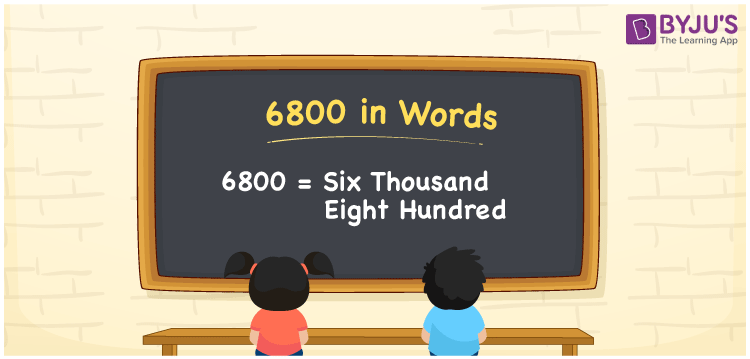# 6800 in Words

We can write 6800 in words as Six thousand eight hundred. We can convert numbers to word form using a place value chart. If you bought a new headset at Rs. 6800, then you can say, “I bought a new headset worth Six thousand eight hundred rupees”. In the same way, we use the word form of cardinal numbers to express a specific price or quantity. In this article, you will learn how to derive the word form of the number 6800 in an easily understandable method.

 6800 in words Six thousand eight hundred Six thousand eight hundred in Numbers 6800

## 6800 in English Words

We generally write numbers in words using the English alphabet. So, we can spell 6800 in English as “Six thousand eight hundred”.## How to Write 6800 in Words?

The number 6800 has four digits so we need to create a place value chart with four columns such that we can define the place values for all the digits. This can be done as shown below.

 Thousands Hundreds Tens Ones 6 8 0 0

Here, ones = 0, tens = 0, hundreds = 8, and thousands = 6.

Thus, we can write the expanded form as:

6 × Thousand + 8 × Hundred + 0 × Ten + 0 × One

= 6 × 1000 + 8 × 100 + 0 × 10 + 0 × 1

= 6000 + 800

= Six thousand + Eight hundred

= Six thousand eight hundred

Hence, 6800 in words = Six thousand eight hundred.

6800 is a natural number that precedes 6801 and succeeds 6799.

6800 in words – Six thousand eight hundred

Is 6800 an odd number? – No

Is 6800 an even number? – Yes

Is 6800 a prime number? – No

Is 6800 a composite number? – Yes

Is 6800 a perfect square number? – No

Is 6800 a perfect cube number? – No

## Frequently Asked Questions on 6800 in Words

Q1

### How do you spell 6800 in English?

The spelling of 6800 in English words is Six thousand eight hundred. So, we can spell the number 6800 as Six thousand eight hundred.
Q2

### How to write 6800 in words on a cheque?

On a cheque, we can write 6800 in words as Six thousand eight hundred rupees only.
Q3

### What number is Six thousand eight hundred in words?

The number 6800 is written as Six thousand eight hundred in words.